A SAS programmer asked how to rearrange elements of a matrix. The rearrangement he wanted was rather complicated: certain blocks of data needed to move relative to other blocks, but the values within each block were to remain unchanged. It turned out that the mathematical operation he needed is called a block transpose. The BTRAN function in SAS/IML performs block-transpose operations, so the complicated rearrangement was easy to implement with a judicious call to the BTRAN function.

This article discusses the block-transpose operation and gives an example. It also shows how a block transpose can conveniently transform wide data into long data and vice versa.

### The block-transpose operation

In general, a block matrix can contain blocks of various sizes. However, the BTRAN function requires that all blocks be the same size. Specifically, suppose A is a block matrix where each block is an n x m matrix. That means that A is an (r n) x (c m) matrix for some whole numbers r and c. The BTRAN function transposes the blocks to create a (c n) x (r m) block matrix.

The idea is more easily explained by a picture. The following image shows a matrix A that is composed of six blocks (of the same size). The block-transpose operation rearranges the blocks but leaves the elements within the blocks unchanged.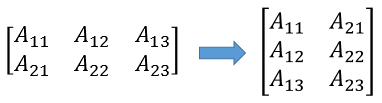### The BTRAN function in SAS/IML

Let's see how the block transpose works on an example in the SAS/IML language. The following statements create a 4 x 12 matrix. I have overlaid some grid lines to help you visualize this matrix as a 2 x 3 block matrix, where each block is 2 x 4.

```proc iml; x = repeat(1:12,2); x = x // (10+x); print x;```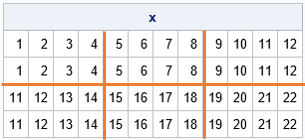You can use the BTRAN function to apply a block transpose. The first argument is the matrix that contains the data. The second and third arguments specify the size of the blocks:

```y = btran(x, 2, 4); /* block size is 2x4 */ print y;```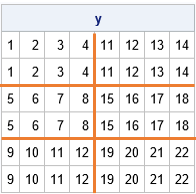The output is a 6 x 8 matrix, visualized as a 3 x 2 block matrix.

### Block transpose for wide-to-long transforms

If the number of rows in the block equals the number of rows in the data, then the BTRAN function stacks columns. In particular, if the block size is n x 1, the BTRAN function stacks multiple variables into a single column, just like the SHAPECOL function. For example, the Sashelp.Iris data contains four variables named SepalLength, SepalWidth, PetalLength, and PetalWidth. The following SAS/IML statements stack the four variables into a single column and create a new column that identifies the name of the original variable:

```proc iml; /* wide to long */ varNames = {"SepalLength" "SepalWidth" "PetalLength" "PetalWidth"}; use Sashelp.Iris; read all var "Species"; /* 150 x 3 */ read all var varNames into X; /* 150 x 4 */ close; n = nrow(X); m = ncol(X); /* n = 150; m = 4 */   ID = repeat(Species, m); /* repeat the vars that are not transposed */ Var = shapecol(repeat(varNames, n), n*m); /* create column that contains variable names */ Value = btran(X, n, 1); /* vector with 150*4 rows */   create test var {ID "Var" "Value"}; append; close;```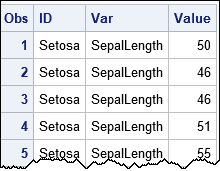### Block transpose for wide-to-long transforms

In a similar way, you can transpose balanced data from long form to wide form. (Recall that "balanced data" means that each group has the same number of observations.) For example, the Sashelp.Iris data contains a Species variable. The first 50 observations have the value 'Setosa', the next 50 have the value 'Versicolor', and the last 50 have the value 'Virginica'. You can use a WHERE clause or a BY statement to analyze each species separately, but suppose you want to create a new data set that contains only 50 observations and has variables named SepalLengthSetosa, SepalLengthVersicolor, SepalLengthVirginica, and so forth for the other variables. The BTRAN function makes this easy: just specify a block size of 50 x 4, as follows.

```/* create pairwise combinations of var names and group levels */ BlockRows = 50; w = btran(X, BlockRows, m); /* 50 x 12 matrix */   s = Species[ do(1, n, BlockRows) ]; /* s = {'Setosa' 'Versicolor', 'Virginica'} */ vNames = rowcatc(expandgrid(s, varNames)); /* combine var names and species */ print vNames, w[c=vNames L="Measurenents (cm)"];```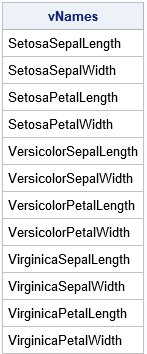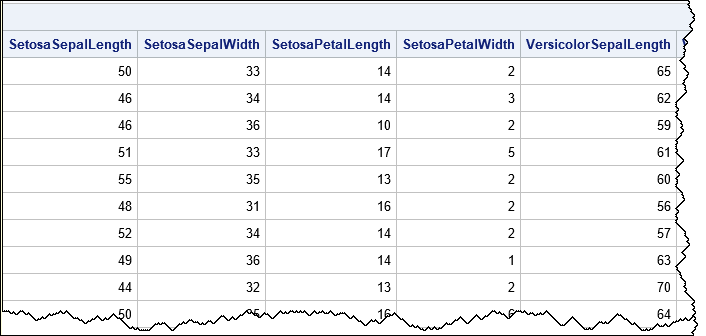In summary, the BTRAN function is a useful function when you need to rearrange blocks of data without changing the values in a block. For balanced designs, you can use the BTRAN function to convert data between wide and long formats.

Share

### About AuthorDistinguished Researcher in Computational Statistics

Rick Wicklin, PhD, is a distinguished researcher in computational statistics at SAS and is a principal developer of PROC IML and SAS/IML Studio. His areas of expertise include computational statistics, simulation, statistical graphics, and modern methods in statistical data analysis. Rick is author of the books Statistical Programming with SAS/IML Software and Simulating Data with SAS.